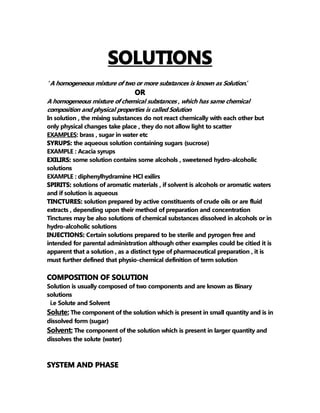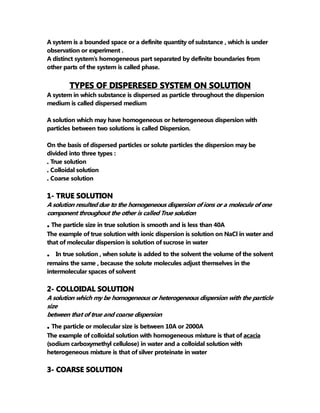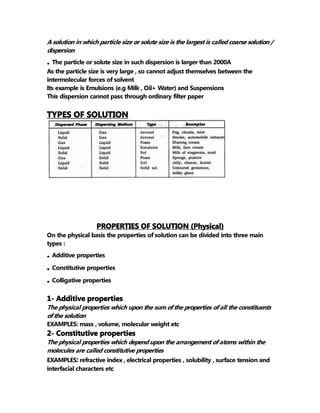Seu SlideShare está sendo baixado. ×

# Pharmaceutical SOLTUIONS by Hemas Mehmood .pdf

Anúncio
Anúncio
Anúncio
Anúncio
Anúncio
Anúncio
Anúncio
Anúncio
Anúncio
Anúncio
AnúncioAnúncio
Anúncio
Carregando em…3
×

1 de 10 Anúncio

# Pharmaceutical SOLTUIONS by Hemas Mehmood .pdf

THE SOLUTIONS ARE THE HIOMOGENOUS MIXTURES OF TWO OR MORE THAN TWO COMPONENTS
THE PHARMACETICAL SOLUTIONS ARE THE SOLUTIONS THATARE TO USED IN THE MANUFACTURING OF THE MEDICINAL PRODCUTS
SUCH MEDICAL RELATED SOLUTIONS ARE CATEGORIZED IN MANY DIFFERENT CLASSES GIVEN ALONG WITH THE DETAILS IN THE SLIDES

THE SOLUTIONS ARE THE HIOMOGENOUS MIXTURES OF TWO OR MORE THAN TWO COMPONENTS
THE PHARMACETICAL SOLUTIONS ARE THE SOLUTIONS THATARE TO USED IN THE MANUFACTURING OF THE MEDICINAL PRODCUTS
SUCH MEDICAL RELATED SOLUTIONS ARE CATEGORIZED IN MANY DIFFERENT CLASSES GIVEN ALONG WITH THE DETAILS IN THE SLIDES

Anúncio
Anúncio

### Pharmaceutical SOLTUIONS by Hemas Mehmood .pdf

1. 1. SOLUTIONS ’ A homogeneous mixture of two or more substances is known as Solution. ’ OR A homogeneous mixture of chemical substances , which has same chemical composition and physical properties is called Solution In solution , the mixing substances do not react chemically with each other but only physical changes take place , they do not allow light to scatter EXAMPLES: brass , sugar in water etc SYRUPS: the aqueous solution containing sugars (sucrose) EXAMPLE : Acacia syrups EXILIRS: some solution contains some alcohols , sweetened hydro-alcoholic solutions EXAMPLE : diphenylhydramine HCl exilirs SPIRITS: solutions of aromatic materials , if solvent is alcohols or aromatic waters and if solution is aqueous TINCTURES: solution prepared by active constituents of crude oils or are fluid extracts , depending upon their method of preparation and concentration Tinctures may be also solutions of chemical substances dissolved in alcohols or in hydro-alcoholic solutions INJECTIONS: Certain solutions prepared to be sterile and pyrogen free and intended for parental administration although other examples could be citied it is apparent that a solution , as a distinct type of pharmaceutical preparation , it is must further defined that physio-chemical definition of term solution COMPOSITION OF SOLUTION Solution is usually composed of two components and are known as Binary solutions i.e Solute and Solvent Solute: The component of the solution which is present in small quantity and is in dissolved form (sugar) Solvent: The component of the solution which is present in larger quantity and dissolves the solute (water) SYSTEM AND PHASE
2. 2. A system is a bounded space or a definite quantity of substance , which is under observation or experiment . A distinct system’s homogeneous part separated by definite boundaries from other parts of the system is called phase. TYPES OF DISPERESED SYSTEM ON SOLUTION A system in which substance is dispersed as particle throughout the dispersion medium is called dispersed medium A solution which may have homogeneous or heterogeneous dispersion with particles between two solutions is called Dispersion. On the basis of dispersed particles or solute particles the dispersion may be divided into three types : . True solution . Colloidal solution . Coarse solution 1- TRUE SOLUTION A solution resulted due to the homogeneous dispersion of ions or a molecule of one component throughout the other is called True solution . The particle size in true solution is smooth and is less than 40A The example of true solution with ionic dispersion is solution on NaCl in water and that of molecular dispersion is solution of sucrose in water . In true solution , when solute is added to the solvent the volume of the solvent remains the same , because the solute molecules adjust themselves in the intermolecular spaces of solvent 2- COLLOIDAL SOLUTION A solution which my be homogeneous or heterogeneous dispersion with the particle size between that of true and coarse dispersion . The particle or molecular size is between 10A or 2000A The example of colloidal solution with homogeneous mixture is that of acacia (sodium carboxymethyl cellulose) in water and a colloidal solution with heterogeneous mixture is that of silver proteinate in water 3- COARSE SOLUTION
3. 3. A solution in which particle size or solute size is the largest is called coarse solution / dispersion . The particle or solute size in such dispersion is larger than 2000A As the particle size is very large , so cannot adjust themselves between the intermolecular forces of solvent Its example is Emulsions (e.g Milk , Oil+ Water) and Suspensions This dispersion cannot pass through ordinary filter paper TYPES OF SOLUTION PROPERTIES OF SOLUTION (Physical) On the physical basis the properties of solution can be divided into three main types : . Additive properties . Constitutive properties . Colligative properties 1- Additive properties The physical properties which upon the sum of the properties of all the constituents of the solution EXAMPLES: mass , volume, molecular weight etc 2- Constitutive properties The physical properties which depend upon the arrangement of atoms within the molecules are called constitutive properties EXAMPLES: refractive index , electrical properties , solubility , surface tension and interfacial characters etc
4. 4. 3- Colligative properties The physical properties of the solution which depend upon the Na of atoms of the solution , are called colligative properties Some important colligative properties are 1. Elevation of B.P 2. Lowering of M.P 3. Depression i n F.P 4. Osmotic pressure The values of colligative properties are approximately same for the solution of the same concentration Types of solutions The solutes (whether they are liquid , solid or gaseous phase) are classified into two types on the basis of their electrical properties: Electrolytes Non - electrolytes Electrolytes Those solutions which are ionized in the solution and conduct electricity are known as electrolytes Such solutions show greater colligative properties as compared to the others with the same concentration Electrolytes are further divided into two classes : Strong electrolytes : Those electrolytes which ionize completely in the solution are called as strong electrolytes EXAMPLES: NaCl , Na2SO4 , HCl etc Weak electrolytes: Weak electrolytes ionize in the solution partially EXAMPLES : Ephedrine , Phenobarbital etc Non - Electrolytes Those solutes which do not ionize in the solutions and cannot conduct electricity are called non-electrolytes EXAMPLES: Sucrose , Urea and Naphthalene etc UNSATURATED SOLUTION : A solution in which more solute is present than the solvent can afford and the solute is not dissolved in the solution completely SATURATED SOLUTION : The one where concentration is at maximum , no more
5. 5. solute is able to dissolve at a given temperature . It represents an equilibrium . The rate of dissolving = The rate of crystallization Salt continues to dissolves ; but crystallizes t the same rate or under those conditions the number of molecules leaving solute = the number of molecules returning to the solid phase i-e solute SUPER-SATURATED SOLUTION: A solution that contain relatively larger amount of solute than that required for saturation , it is prepared by heating and adding more and more solute CONCENTRATION EXPRESSION The amount of solute present in a given amount of the solution is called as concentration expression A solution containing relatively lower amount of solute is known as Dilute solution while A solution containing relatively higher amount of solute is known as Concentrated solution Concentration of solution may either be expressed in terms of quantity of solute in a definite volume in solution or as the quantity of solute in a definite mass of solvent or solution There are several ways of expressing concentration of the solution , some of them are as follow : 1- Percentage Expression 2- Molarity 3- Normality 4- Molality 5- Mole fraction 6- Parts per million 7- Percent by volume 7- Percent by weight 8- Percent weight in volume 9- Milligram percent 1- Percentage Expression : It is defined as the specific amount of solutes present in 100 gm of solution Following are three types of percentage expression : (a) %age w/w : It is the weight of solute as present in total weight of solution
6. 6. %age of solute = weight of solute * 100 weight of solution Example : 10% w/w aqueous solution of glycerine means 10g of glycerine dissolved in enough water to make it 1000 grams (b) %age v/v : It is the volume of solute as present in total volume of solution %age of solute = volume of solute * 100 Volume of solution Example : 10% v/v aqueous ehthanoic solution means 10 ml of ethanol dissolved in enough water o make it 1 liter (c) %age v/w : It is the volume of solute as present in total weight of solution %age of solute = volume of solute * 100 weight of solution Example : 10% v/w aqueous glycerine means 10 ml of glycerine dissolved in enough water to make it 1000 grams of solution (d) %age w/ v : It is the weight of solute as present in total volume of solution %age of solute = weight of solute * 100 volume of solution Example : 10% w/v aqueous NaCl solution means 10 grams dissolved in enough water to make it 1 liter 2- Molarity and Normality : all solution of same molarity contains the same no. of solute molecules in definite volume of solution In case of more than one solute , it may have different molar concentration of every solute EXAMPLE : MOLARITY: A solution can be 0.001M phenobarbital and 0.1M NaCl w.r.t 1 liter of solution prepared by 0.001 mole of phenobarbital (0.001 mol * 263 g/mol = 0.2323g) and 0.1 mole of NaCl (0.1 mol * 58.45 g/mol = 5.845g ) to enough water to make 1000 ml of solution NORMALITY: A molar solution of NaCl is 1M w.r.t both Na+ and Cl- ions , whereas molar solution of Na2CO3 is 1M w.r.t Na+ is 2M and that of carbonate is 1M A molar solution of NaCl is 1 normal with respect to both ,however, that of Na2CO3 is 2M w.r.t both Molarity : It is defined as the number of moles of solutes dissolved per liter(1l= 1000g) of solution Molarity= no. of moles of solutes
7. 7. Volume of solution (in liters) Where no. of moles = given weight Molar mass It is represented by M and its units are mol/liters. EXAMPLE : Determine the molarity of 41.50g FeSO4 ? Moles of FeSO4 = grams of FeSO4 = 41.50 = 0.2732 Mol. Weight 151.9 M = 0.2732 = 0.2732 1 liter Normality : The number of grams equivalent of solute present per liter of solution Normality : no. of grams equivalent of solute Volume of solution (in liters) Where no. of gm equivalent = weight of substance Equivalent weight Equivalent weight : no. of parts by weight of the substance combines with one part with weight of replacement H+ or OH- or number of +ive charges on element Equivalent weight = molecular weight Acidity or basicity EXAMPLE: Calculate the normality of H2SO4 solution where 98g of H2SO4 is dissolved in 500 liters? Equivalent weight of H2SO4 = 49 No. of grams equivalent of H2SO4 = 98/49 = 2 Volume of solution = 500cm3 = 0.5 liters Normality = 2/0.5 = 4N DISADVANTAGES: Molarity and normality has a disadvantage of changing of value with the temperature Because the volume is a temperature dependent value because of the contraction and expansion of liquid. MOLALITY
8. 8. The number of moles of solute per kilogram of solvent it is represented by m and its unit is mol/kg m = no. of moles Kilogram of solvent A molal solution is prepared in terms of weight units and does not have disadvantages just discussed above (temperature) therefore , molal concentration appear more frequent than molarity and normality in theoretic studies EXAMPLE : 1- Calculate the molality of 6.4 grams of methanol dissolved in 50 moles of water? a) 0.040 m b) 0.22 m c) 0.064 m d) 0.11 m m =moles of solute = 6.4/32 Kg of solvent 50*18 2- Find the molality Grams of solution = volume * density 1000*1.0375= 1037.5 Grams of solvent = grams of solution - grams of FeSO4 1037.5- 41.5 = 996 g Molality = moles of FeSO4 = o.2732 = 0.2743 kg of solvent 0.996 3- MOLE FRACTION The ratio of no. of moles of one constituents to no. of moles of all the constituents in the solution is called mole fraction It is denoted by “X” Mole fraction is frequently used in experimentation involving theoretical consideration It gives a measure of each constituent in a solution It is expressed for a system of two constituents which are X1 is the MF of solvent X2 is the MF of solute n1 is the no. of moles of solvent n2 is the no. of moles of solute
9. 9. X1 = n1 X2 = n2 n1 + n2 n1+n2 X1 + X2 = 1 Sum of MF of solute and solvent is always equals to unity So mole percentage will always be equal to 100% In a solution containing 0.01 mole of solute and 0.04 moles of solvent the MF of X2 will be 0.20 We know that the MF of two constituents must be equal to 1 so the MF of solvent will be 0.80 The mole percent of solute will be 20% and that of solvent will be 80% The manner in which MF is defined allows one to express the relationship between the no. of solute and solvent molecules in a simple direct way It means two out of every 10 molecules in a solution are solute molecules and that many of the solute and solvent properties are directly related to the MF of the solution The partial vapor pressure above a solution brought about the pressure of volatile solute = the vapor pressure of pure solute * the MF of the solute in solution EXAMPLE : Moles of water = 996 = 55.5 moles 18.02 Moles of FeSO4 = 41.50 = 0.2732 151.9 MF of FeSO4 = 0.2732 = 0.049 0.2732+55.5 MF of water = 55.5 = 0.0951 0.2732+55.5 Note that X1 + X2 = 1 So mole percent will also 100% 5- PARTS PER MILLION/BILLION it is another way of expressing concentration in a particular way that very dilute solution can also be expressed e.g to describe the impurities in a solution ppm denotes the amount of given substance in total amount of 1000000 of solution e.g one mg per kg i.e 1 part in 106 ppb denotes the amount of given substance in a total amount 1000000000 of solution e.g 0.001 mg per kg of solution i.e 1 part in 109
10. 10. Ppm/ppb = mass of A in solution * 106/109 Total mass of solution EXAMPLE : if 3.6 mg of Hg is detected of 200g sample of H2O from lake Saif-ul-Malook what is its ppm concentration ? Ppm = 0.0036 * 106 = 18ppm 200 CHANGING OF MOLARITY INTO MOLALITY